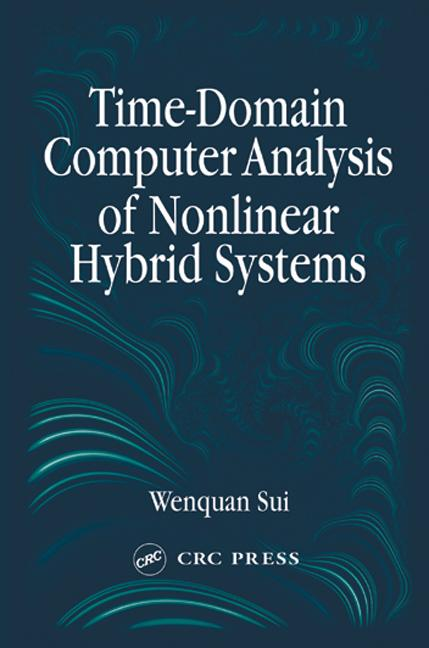Time-Domain Computer Analysis of Nonlinear Hybrid Systems

1st Edition

CRC Press

416 pages | 236 B/W Illus.

Purchasing Options:\$ = USD
Hardback: 9780849313967
pub: 2001-11-06
SAVE ~\$48.00
Currently out of stock
\$240.00
\$192.00
x
eBook (VitalSource) : 9781315220284
pub: 2018-10-08
from \$28.98

FREE Standard Shipping!

Description

The analysis of nonlinear hybrid electromagnetic systems poses significant challenges that essentially demand reliable numerical methods. In recent years, research has shown that finite-difference time-domain (FDTD) cosimulation techniques hold great potential for future designs and analyses of electrical systems.

Time-Domain Computer Analysis of Nonlinear Hybrid Systems summarizes and reviews more than 10 years of research in FDTD cosimulation. It first provides a basic overview of the electromagnetic theory, the link between field theory and circuit theory, transmission line theory, finite-difference approximation, and analog circuit simulation. The author then extends the basic theory of FDTD cosimulation to focus on techniques for time-domain field solving, analog circuit analysis, and integration of other lumped systems, such as n-port nonlinear circuits, into the field-solving scheme.

The numerical cosimulation methods described in this book and proven in various applications can effectively simulate hybrid circuits that other techniques cannot. By incorporating recent, new, and previously unpublished results, this book effectively represents the state of the art in FDTD techniques. More detailed studies are needed before the methods described are fully developed, but the discussions in this book build a good foundation for their future perfection.

Reviews

"…Easy to read, concise, and complete."

-Alfy Riddle, IEEE Microwave Magazine

INTRODUCTION

Introduction

Electromagnetic Systems

Hybrid Electromagnetic Systems

Organization of the Book

ELECTROMAGNETIC FIELD THEORY

Introduction

Electromagnetic Theory

Example of Solving Electromagnetic Field Distribution

CIRCUIT EQUIVALENCE AND TRANSMISSION LINE THEORY

Circuit Theory as Field Approximation

Transmission Line Theory

Scattering Parameters of an n-Port Network

FINITE-DIFFERENCE FORMULATION

Introduction

Finite-Difference Method

System Solution and Stability Condition

SOLVING ELECTROMAGNETIC FIELDS IN THE TIME DOMAIN-FDTD METHOD

Introduction

Finite-Difference Time-Domain (FDTD) Method

Issues of FDTD Numerical Implementation

Examples of FDTD Application

CIRCUIT FORMULATION AND COMPUTER SIMULATION

Introduction

Constitutive Relation of Devices

Modified Nodal Formulation of Circuit Simulation

Transient Analysis of Linear Circuit

Nonlinear Device Models in Circuit Simulation

Newton Method for Solving System with Nonlinear Devices

Timestep Control in Transient Simulation

FORMULATION FOR HYBRID SYSTEM SIMULATION IN THE TIME DOMAIN

Introduction

Maxwell's Equations and Supplemental Current Equations

Hybrid Circuit Simulation with Lumped Elements

Electron Beam in FDTD Simulation

INTERFACING FDTD FIELD SOLVER WITH LUMPED SYSTEMS

Introduction

Linking FDTD Method with a SPICE-Like Circuit Simulator

Modeling a Multiport S-Parameter Network in FDTD

Multiport Behavioral Model in FDTD

Examples of General Hybrid System Cosimulation

SIMULATION OF HYBRID ELECTROMAGNETIC SYSTEMS

Introduction

FDTD Characterization and De-Embedding

Examples of Hybrid System Cosimulation

Analysis of Packaging Structure with On-Chip Circuit

OPTICAL DEVICE SIMULATION IN FDTD

Introduction

Active Gain Media in VCSEL

FDTD Formulation for Systems with Nonlinear Gain Media

FDTD Analysis of VCSEL Structure

Cosimulation for VCSEL Source and Other Circuits

Appendix I: Vector Differential Operators and Vector Identities

Appendix II: Laplace Transformation

REFERENCES

Index